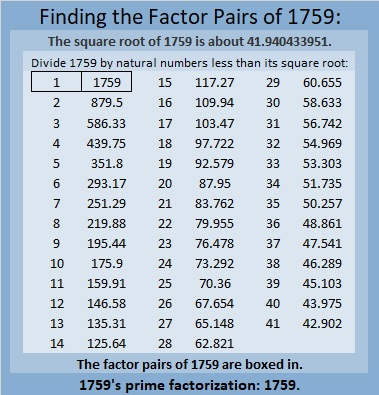# 1759 Pie Over Two

### Today’s Puzzle:

Today in the United States many students will celebrate pi day by eating pie. Today’s puzzle looks a little like a pie that has been cut in half, so I’m calling it pie over two, abbreviated as “π/2”.

Write the numbers 1 to 12 in the first column and again in the top row so that those numbers and the given clues make a multiplication table. Be sure to use logic every step of the way.### Factors of 1759:

• 1759 is a prime number.
• Prime factorization: 1759 is prime.
• 1759 has no exponents greater than 1 in its prime factorization, so √1759 cannot be simplified.
• The exponent in the prime factorization is 1. Adding one to that exponent we get (1 + 1) = 2. Therefore 1759 has exactly 2 factors.
• The factors of 1759 are outlined with their factor pair partners in the graphic below.How do we know that 1759 is a prime number? If 1759 were not a prime number, then it would be divisible by at least one prime number less than or equal to √1759. Since 1759 cannot be divided evenly by 2, 3, 5, 7, 11, 13, 17, 19, 23, 29, 31, 37, or 41, we know that 1759 is a prime number.

### More About the Number 1759:

Like every other odd number, 1759 is the difference of two squares:
880² – 879² = 1759.

OEIS.org informs us that 1759 is only the 17th Eisenstein-Mersenne prime number.